The Idea Behind Gradient Descent 梯度下降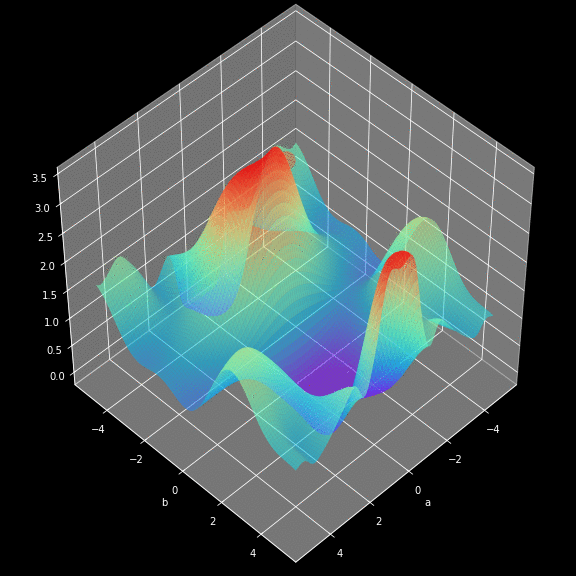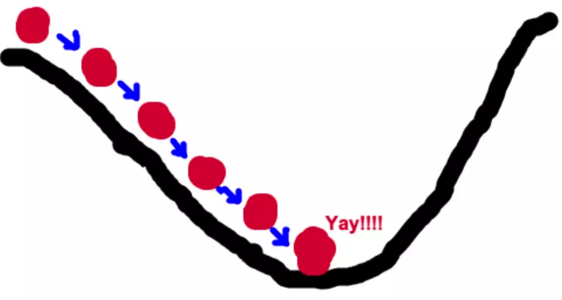• 假设此时山上的浓雾很大，下山的路无法确定;

• 假设你摔不死！

• 你只能利用自己周围的信息去找到下山的路径。

• 以你当前的位置为基准，寻找这个位置最陡峭的方向，从这个方向向下走。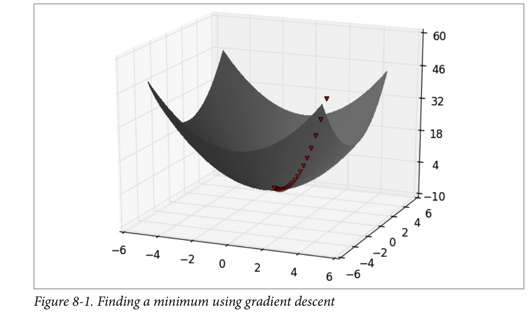Gradient is the vector of partial derivatives

One approach to maximizing a function is to

• pick a random starting point,

• take a small step in the direction of the gradient, and

• repeat with a new staring point.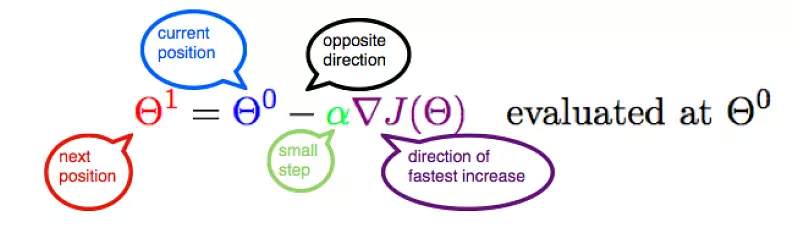Let’s represent parameters as $\Theta$, learning rate as $\alpha$, and gradient as $\bigtriangledown J(\Theta)$,

To the find the best model is an optimization problem

• “minimizes the error of the model”

• “maximizes the likelihood of the data.”

We’ll frequently need to maximize (or minimize) functions.

• to find the input vector v that produces the largest (or smallest) possible value.

A simple mathematical intuition behind one of the commonly used optimisation algorithms in Machine Learning.

https://www.douban.com/note/713353797/

The cost or loss function:

$$Cost = \frac{1}{N} \sum_{i = 1}^N (Y’ -Y)^2$$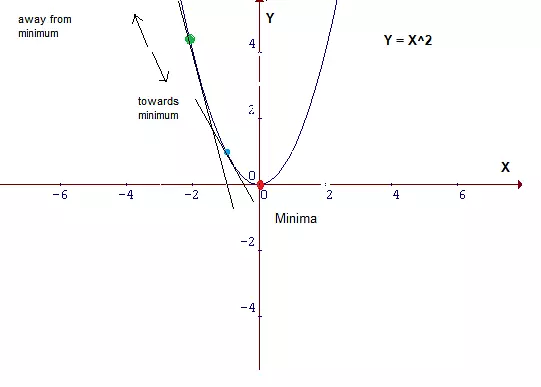Parameters with small changes: $$m_1 = m_0 - \delta m, b_1 = b_0 - \delta b$$

The cost function J is a function of m and b:

$$J_{m, b} = \frac{1}{N} \sum_{i = 1}^N (Y’ -Y)^2 = \frac{1}{N} \sum_{i = 1}^N Error_i^2$$

$$\frac{\partial J}{\partial m} = 2 Error \frac{\partial}{\partial m}Error$$

$$\frac{\partial J}{\partial b} = 2 Error \frac{\partial}{\partial b}Error$$

Let’s fit the data with linear regression:

$$\frac{\partial}{\partial m}Error = \frac{\partial}{\partial m}(Y’ - Y) = \frac{\partial}{\partial m}(mX + b - Y)$$

Since $X, b, Y$ are constant:

$$\frac{\partial}{\partial m}Error = X$$

$$\frac{\partial}{\partial b}Error = \frac{\partial}{\partial b}(Y’ - Y) = \frac{\partial}{\partial b}(mX + b - Y)$$

Since $X, m, Y$ are constant:

$$\frac{\partial}{\partial m}Error = 1$$

Thus:

$$\frac{\partial J}{\partial m} = 2 * Error * X$$ $$\frac{\partial J}{\partial b} = 2 * Error$$

Let’s get rid of the constant 2 and multiplying the learning rate $\alpha$, who determines how large a step to take:

$$\frac{\partial J}{\partial m} = Error * X * \alpha$$ $$\frac{\partial J}{\partial b} = Error * \alpha$$

Since $m_1 = m_0 - \delta m, b_1 = b_0 - \delta b$:

$$m_1 = m_0 - Error * X * \alpha$$

$$b_1 = b_0 - Error * \alpha$$

Notice that the slope b can be viewed as the beta value for X = 1. Thus, the above two equations are in essence the same.

Let’s represent parameters as $\Theta$, learning rate as $\alpha$, and gradient as $\bigtriangledown J(\Theta)$, we have:

$$\Theta_1 = \Theta_0 - \alpha \bigtriangledown J(\Theta)$$Hence,to solve for the gradient, we iterate through our data points using our new $m$ and $b$ values and compute the partial derivatives.

• the slope of our cost function at our current position

• the direction we should move to update our parameters.

• The size of our update is controlled by the learning rate.

import numpy as np

# Size of the points dataset.
m = 20
# Points x-coordinate and dummy value (x0, x1).
X0 = np.ones((m, 1))
X1 = np.arange(1, m+1).reshape(m, 1)
X = np.hstack((X0, X1))
# Points y-coordinate
y = np.array([3, 4, 5, 5, 2, 4, 7, 8, 11, 8, 12,
11, 13, 13, 16, 17, 18, 17, 19, 21]).reshape(m, 1)

# The Learning Rate alpha.
alpha = 0.01

def error_function(theta, X, y):
'''Error function J definition.'''
diff = np.dot(X, theta) - y
return (1./2*m) * np.dot(np.transpose(diff), diff)

'''Gradient of the function J definition.'''
diff = np.dot(X, theta) - y
return (1./m) * np.dot(np.transpose(X), diff)

theta = np.array([1, 1]).reshape(2, 1)
theta = theta - alpha * gradient
return theta

# source：https://www.jianshu.com/p/c7e642877b0e

optimal = gradient_descent(X, y, alpha)
print('Optimal parameters Theta:', optimal, optimal)
print('Error function:', error_function(optimal, X, y)[0,0])

Optimal parameters Theta: 0.5158328581734093 0.9699216324486175
Error function: 405.98496249324046If f is a function of one variable, its derivative at a point x measures how f(x) changes when we make a very small change to x.

It is defined as the limit of the difference quotients:

def difference_quotient(f, x, h):
return (f(x + h) - f(x)) / h


For many functions it’s easy to exactly calculate derivatives.

For example, the square function:

    def square(x):
return x * x


has the derivative:

    def derivative(x):
return 2 * x

def square(x):
return x * x

def derivative(x):
return 2 * x

derivative_estimate = lambda x: difference_quotient(square, x, h=0.00001)

def sum_of_squares(v):
"""computes the sum of squared elements in v"""
return sum(v_i ** 2 for v_i in v)

# plot to show they're basically the same
import matplotlib.pyplot as plt
x = range(-10,10)
plt.plot(x, list(map(derivative, x)), 'rx')           # red  x
plt.plot(x, list(map(derivative_estimate, x)), 'b+')  # blue +
plt.show()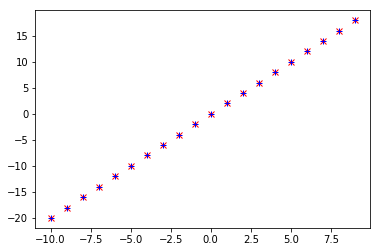When f is a function of many variables, it has multiple partial derivatives.

def partial_difference_quotient(f, v, i, h):
# add h to just the i-th element of v
w = [v_j + (h if j == i else 0)
for j, v_j in enumerate(v)]
return (f(w) - f(v)) / h

return [partial_difference_quotient(f, v, i, h)
for i, _ in enumerate(v)]


def step(v, direction, step_size):
"""move step_size in the direction from v"""
return [v_i + step_size * direction_i
for v_i, direction_i in zip(v, direction)]

return [2 * v_i for v_i in v]

from collections import Counter
from linear_algebra import distance, vector_subtract, scalar_multiply
from functools import reduce
import math, random

print("using the gradient")

# generate 3 numbers
v = [random.randint(-10,10) for i in range(3)]
print(v)
tolerance = 0.0000001

n = 0
while True:
if n%50 ==0:
print(v, sum_of_squares(v))
if distance(next_v, v) < tolerance:     # stop if we're converging
break
v = next_v                              # continue if we're not
n += 1

print("minimum v", v)
print("minimum value", sum_of_squares(v))

using the gradient
[-4, 10, 6]
[-4, 10, 6] 152
[-1.4566787203484681, 3.641696800871171, 2.1850180805227026] 20.15817249600249
[-0.5304782235790126, 1.3261955589475318, 0.7957173353685193] 2.6733678840696777
[-0.19318408497395115, 0.482960212434878, 0.28977612746092685] 0.35454086152861664
[-0.07035178642288623, 0.17587946605721566, 0.10552767963432938] 0.04701905160246833
[-0.025619987555179666, 0.0640499688879492, 0.03842998133276951] 0.006235645742111834
[-0.0093300226718057, 0.023325056679514268, 0.013995034007708552] 0.0008269685690358806
[-0.0033977113715970304, 0.008494278428992584, 0.005096567057395551] 0.00010967220436445803
[-0.0012373434632228497, 0.003093358658057131, 0.0018560151948342769] 1.4544679036813049e-05
[-0.0004506029731597509, 0.0011265074328993788, 0.0006759044597396269] 1.9289088744938724e-06
[-0.00016409594058189027, 0.00041023985145472635, 0.00024614391087283554] 2.558110382968256e-07
[-5.975876618530154e-05, 0.00014939691546325416, 8.963814927795238e-05] 3.3925546291900725e-08
[-2.1762330764102098e-05, 5.440582691025532e-05, 3.264349614615315e-05] 4.499190882718763e-09
[-7.925181032313087e-06, 1.9812952580782747e-05, 1.1887771548469638e-05] 5.96680696751885e-10
[-2.8861106411699463e-06, 7.215276602924873e-06, 4.32916596175492e-06] 7.913152901420692e-11
minimum v [-1.6064572436336709e-06, 4.0161431090841815e-06, 2.409685865450507e-06]
minimum value 2.4516696318419405e-11


### Choosing the Right Step Size¶

Although the rationale for moving against the gradient is clear,

• how far to move is not.

• Indeed, choosing the right step size is more of an art than a science.

Methods:

1. Using a fixed step size

2. Gradually shrinking the step size over time

3. At each step, choosing the step size that minimizes the value of the objective function

step_sizes = [100, 10, 1, 0.1, 0.01, 0.001, 0.0001, 0.00001]


It is possible that certain step sizes will result in invalid inputs for our function.

So we’ll need to create a “safe apply” function

• returns infinity for invalid inputs:

• which should never be the minimum of anything

def safe(f):
"""define a new function that wraps f and return it"""
def safe_f(*args, **kwargs):
try:
return f(*args, **kwargs)
except:
return float('inf')  # this means "infinity" in Python
return safe_f


### Putting It All Together¶

• target_fn that we want to minimize

For example, the target_fn could represent the errors in a model as a function of its parameters,

To choose a starting value for the parameters theta_0.

def minimize_batch(target_fn, gradient_fn, theta_0, tolerance=0.000001):
"""use gradient descent to find theta that minimizes target function"""

step_sizes = [100, 10, 1, 0.1, 0.01, 0.001, 0.0001, 0.00001]

theta = theta_0                           # set theta to initial value
target_fn = safe(target_fn)               # safe version of target_fn
value = target_fn(theta)                  # value we're minimizing

while True:
for step_size in step_sizes]

# choose the one that minimizes the error function
next_theta = min(next_thetas, key=target_fn)
next_value = target_fn(next_theta)

# stop if we're "converging"
if abs(value - next_value) < tolerance:
return theta
else:
theta, value = next_theta, next_value

# minimize_batch"
v = [random.randint(-10,10) for i in range(3)]
print("minimum v", v)
print("minimum value", sum_of_squares(v))

minimum v [0.0009304595970494407, -0.001196305196206424, -0.00026584559915698326]
minimum value 2.367575066803034e-06


Sometimes we’ll instead want to maximize a function, which we can do by minimizing its negative

def negate(f):
"""return a function that for any input x returns -f(x)"""
return lambda *args, **kwargs: -f(*args, **kwargs)

def negate_all(f):
"""the same when f returns a list of numbers"""
return lambda *args, **kwargs: [-y for y in f(*args, **kwargs)]

return minimize_batch(negate(target_fn),
theta_0,
tolerance)


Using the batch approach, each gradient step requires us to make a prediction and compute the gradient for the whole data set, which makes each step take a long time.

• The predictive error on the whole data set is simply the sum of the predictive errors for each data point.

When this is the case, we can instead apply a technique called stochastic gradient descent

• which computes the gradient (and takes a step) for only one point at a time.

• It cycles over our data repeatedly until it reaches a stopping point.

During each cycle, we’ll want to iterate through our data in a random order:

def in_random_order(data):
"""generator that returns the elements of data in random order"""
indexes = [i for i, _ in enumerate(data)]  # create a list of indexes
random.shuffle(indexes)                    # shuffle them
for i in indexes:                          # return the data in that order
yield data[i]


This approach avoids circling around near a minimum forever

• whenever we stop getting improvements we’ll decrease the step size and eventually quit.

def minimize_stochastic(target_fn, gradient_fn, x, y, theta_0, alpha_0=0.01):
data = list(zip(x, y))
theta = theta_0                             # initial guess
alpha = alpha_0                             # initial step size
min_theta, min_value = None, float("inf")   # the minimum so far
iterations_with_no_improvement = 0

# if we ever go 100 iterations with no improvement, stop
while iterations_with_no_improvement < 100:
value = sum( target_fn(x_i, y_i, theta) for x_i, y_i in data )

if value < min_value:
# if we've found a new minimum, remember it
# and go back to the original step size
min_theta, min_value = theta, value
iterations_with_no_improvement = 0
alpha = alpha_0
else:
# otherwise we're not improving, so try shrinking the step size
iterations_with_no_improvement += 1
alpha *= 0.9

# and take a gradient step for each of the data points
for x_i, y_i in in_random_order(data):

return min_theta

def maximize_stochastic(target_fn, gradient_fn, x, y, theta_0, alpha_0=0.01):
return minimize_stochastic(negate(target_fn),
x, y, theta_0, alpha_0)

print("using minimize_stochastic_batch")

x = list(range(101))
y = [3*x_i + random.randint(-10, 20) for x_i in x]
theta_0 = random.randint(-10,10)
v = minimize_stochastic(sum_of_squares, sum_of_squares_gradient, x, y, theta_0)

print("minimum v", v)
print("minimum value", sum_of_squares(v))



Scikit-learn has a Stochastic Gradient Descent module http://scikit-learn.org/stable/modules/sgd.html